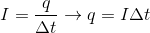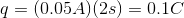# AP Physics B : Understanding Current

## Example Questions

### Example Question #3 : Electric Circuits

A charge travels along a copper wire that has a resistance of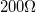. It takes two seconds for the charge to travel along the wire. The potential difference in the wire is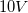. What is the charge of the particle?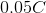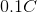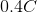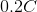Explanation:

To solve this question you need to use the Ohm’s law and the definition of an electric current. Ohm’s law states that voltage is the product of current and resistance: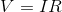The definition of electric current is given by the charge per unit time: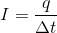Using these equations it is possible to solve for the charge. The trick is to first solve for the current using Ohm’s law and the given voltage and resistance.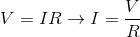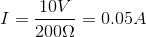Now that we know the current and the time, we can solve for the charge using our second equation.